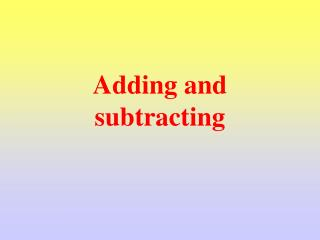DownloadDownload PresentationTélécharger la présentation- - - - - - - - - - - - - - - - - - - - - - - - - - - E N D - - - - - - - - - - - - - - - - - - - - - - - - - - -
##### Presentation Transcript

2. Learning Objective I am able to rapidly recall my number bonds.

3. Shoot the Sherriff

4. Learning Objective I am able to rapidly recall my number bonds.

5. Learning Objective I am able to add up and subtract using a number of different methods.

6. How can I add these numbers up. 39 + 10 = 49 Add on 10 to the Tens columns

7. How can I add these numbers up. 39 + 9 = 48 Add on 10 and take away 1

8. How can I add these numbers up. 44 + 9 = 53 Add on 10 and take away 1

9. How can I add these numbers up. 44 + 11 = 55 Add on 10 and add 1

10. Add 10 12 23 51 26

11. Add 11 12 23 51 26

12. Take – away 11 12 23 51 26

13. Add 9 12 23 51 26

14. Add 9 12 23 51 26

15. Take away 9 12 23 51 26

16. Learning Objective I am able to add up and subtract using a number of different methods.

17. Plenary Today we practised our number bonds. You were able to add and subtract using different strategies.

18. Simple Problem If 12 + 15 = 27 What does 15 + 12 = ? What does 27 – 12 = ? What does 27 – 12 = ?